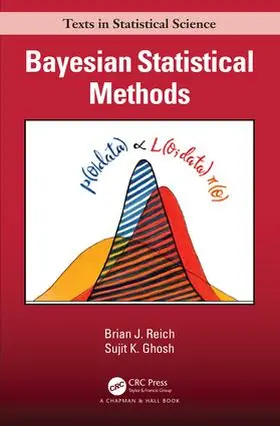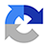# Reich / GhoshBayesian Statistical Methods

1. Auflage 2019, 0 Seiten, Gebunden, Format (B × H): 158 mm x 238 mm, Gewicht: 574 g Reihe: Chapman & Hall/CRC Texts in Statistical Science
ISBN: 978-0-8153-7864-8
Verlag: Taylor & Francis Inc

## Reich / GhoshBayesian Statistical Methods

Bayesian Statistical Methods provides data scientists with the foundational and computational tools needed to carry out a Bayesian analysis. This book focuses on Bayesian methods applied routinely in practice including multiple linear regression, mixed effects models and generalized linear models (GLM). The authors include many examples with complete R code and comparisons with analogous frequentist procedures.
In addition to the basic concepts of Bayesian inferential methods, the book covers many general topics:

Computational methods including Markov chain Monte Carlo (MCMC)

Model-comparison and goodness-of-fit measures, including sensitivity to priors

Frequentist properties of Bayesian methods

Case studies covering advanced topics illustrate the flexibility of the Bayesian approach:

Semiparametric regression

Handling of missing data using predictive distributions

Priors for high-dimensional regression models

Computational techniques for large datasets

Spatial data analysis

The advanced topics are presented with sufficient conceptual depth that the reader will be able to carry out such analysis and argue the relative merits of Bayesian and classical methods. A repository of R code, motivating data sets, and complete data analyses are available on the book’s website.
Brian J. Reich, Associate Professor of Statistics at North Carolina State University, is currently the editor-in-chief of the Journal of Agricultural, Biological, and Environmental Statistics and was awarded the LeRoy & Elva Martin Teaching Award.
Sujit K. Ghosh, Professor of Statistics at North Carolina State University, has over 22 years of research and teaching experience in conducting Bayesian analyses, received the Cavell Brownie mentoring award, and served as the Deputy Director at the Statistical and Applied Mathematical Sciences Institute.

## Weitere Infos & Material

1. Basics of Bayesian Inference
Probability background
Univariate distributions
Discrete distributions
Continuous distributions
Multivariate distributions
Marginal and conditional distributions
Bayes' Rule
Discrete example of Bayes' Rule
Continuous example of Bayes' Rule
Introduction to Bayesian inference
Summarizing the posterior
Point estimation
Univariate posteriors
Multivariate posteriors
The posterior predictive distribution
Exercises

2. From Prior Information to Posterior Inference
Conjugate Priors
Beta-binomial model for a proportion
Poisson-gamma model for a rate
Normal-normal model for a mean
Normal-inverse gamma model for a variance
Natural conjugate priors
Normal-normal model for a mean vector
Normal-inverse Wishart model for a covariance matrix
Mixtures of conjugate priors
Improper Priors
Objective Priors
Jeffreys prior
Reference Priors
Maximum Entropy Priors
Empirical Bayes
Penalized complexity priors
Exercises

3. Computational approaches
Deterministic methods
Maximum a posteriori estimation
Numerical integration
Bayesian Central Limit Theorem (CLT)
Markov Chain Monte Carlo (MCMC) methods
Gibbs sampling
Metropolis-Hastings (MH) sampling
MCMC software options in R
Diagnosing and improving convergence
Selecting initial values
Convergence diagnostics
Improving convergence
Dealing with large datasets
Exercises

4. Linear models
Analysis of normal means
One-sample/paired analysis
Comparison of two normal means
Linear regression
Jeffreys prior
Gaussian prior
Continuous shrinkage priors
Predictions
Example: Factors that affect a home's microbiome
Generalized linear models
Binary data
Count data
Example: Logistic regression for NBA clutch free throws
Example: Beta regression for microbiome data
Random effects
Flexible linear models
Nonparametric regression
Heteroskedastic models
Non-Gaussian error models
Linear models with correlated data
Exercises

5. Model selection and diagnostics
Cross validation
Hypothesis testing and Bayes factors
Stochastic search variable selection
Bayesian model averaging
Model selection criteria
Goodness-of-fit checks
Exercises

6. Case studies using hierarchical modeling
Overview of hierarchical modeling
Case study: Species distribution mapping via data fusion
Case study: Tyrannosaurid growth curves
Case study: Marathon analysis with missing data

7. Statistical properties of Bayesian methods
Decision theory
Frequentist properties
Asymptotics
Simulation studies
Exercises

Appendices
Probability distributions
Univariate discrete
Multivariate discrete
Univariate continuous
Multivariate continuous
List of conjugacy pairs
Derivations
Normal-normal model for a mean
Normal-normal model for a mean vector
Normal-inverse Wishart model for a covariance matrix
Jeffreys' prior for a normal model
Jeffreys' prior for multiple linear regression
Convergence of the Gibbs sampler
Marginal distribution of a normal mean under Jeffreys’ prior
Marginal posterior of the regression coefficients under Jeffreys prior
Proof of posterior consistency
Computational algorithms
Integrated nested Laplace approximation (INLA)
Hamiltonian Monte Carlo (HMC)
Slice sampling
Software comparison
Example - Simple linear regression
Example - Random slopes model

Reich, Brian J. (N.C. State University)
Brian J. Reich, Associate Professor of Statistics at North Carolina State University, is currently the editor-in-chief of the Journal of Agricultural, Biological, and Environmental Statistics and was awarded the LeRoy & Elva Martin Teaching Award.
Sujit K. Ghosh, Professor of Statistics at North Carolina State University, has over 22 years of research and teaching experience in conducting Bayesian analyses, received the Cavell Brownie mentoring award, and served as the Deputy Director at the Statistical and Applied Mathematical Sciences Institute

## Ihre Fragen, Wünsche oder Anmerkungen

Ihre Nachricht*
Wie möchten Sie kontaktiert werden?
Anrede*
Titel
Vorname*
Nachname*